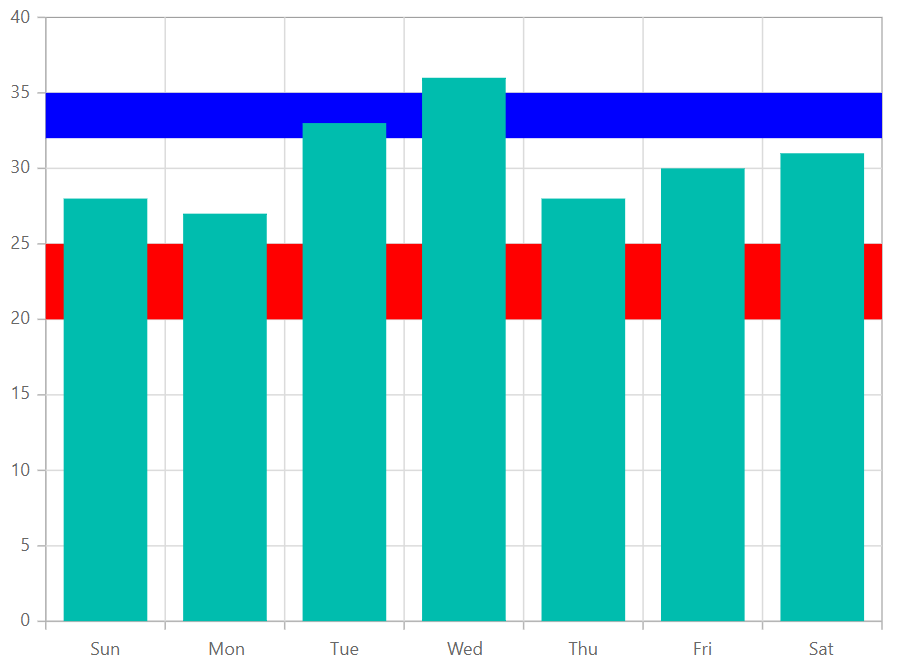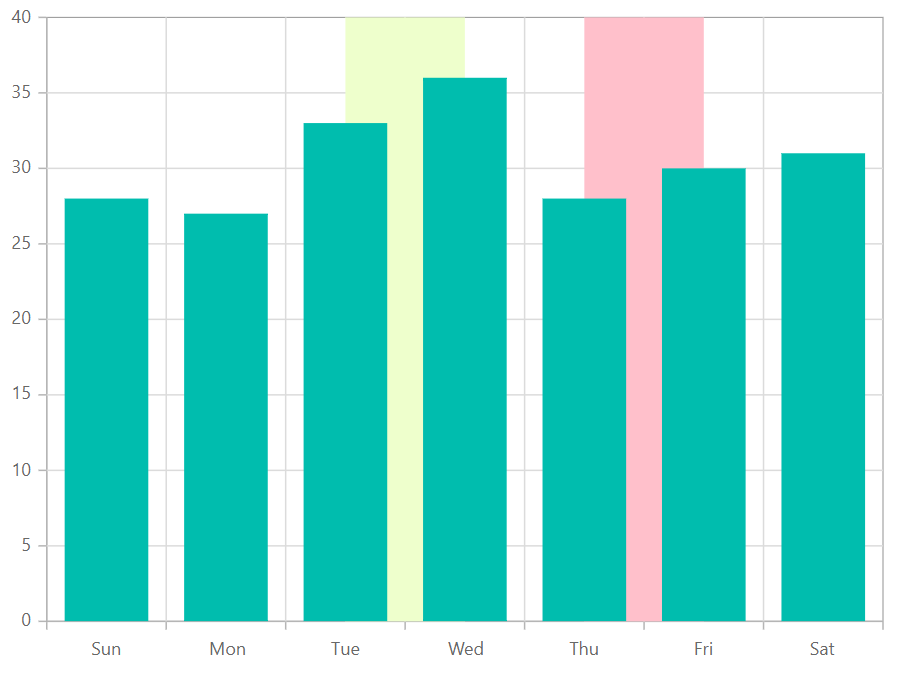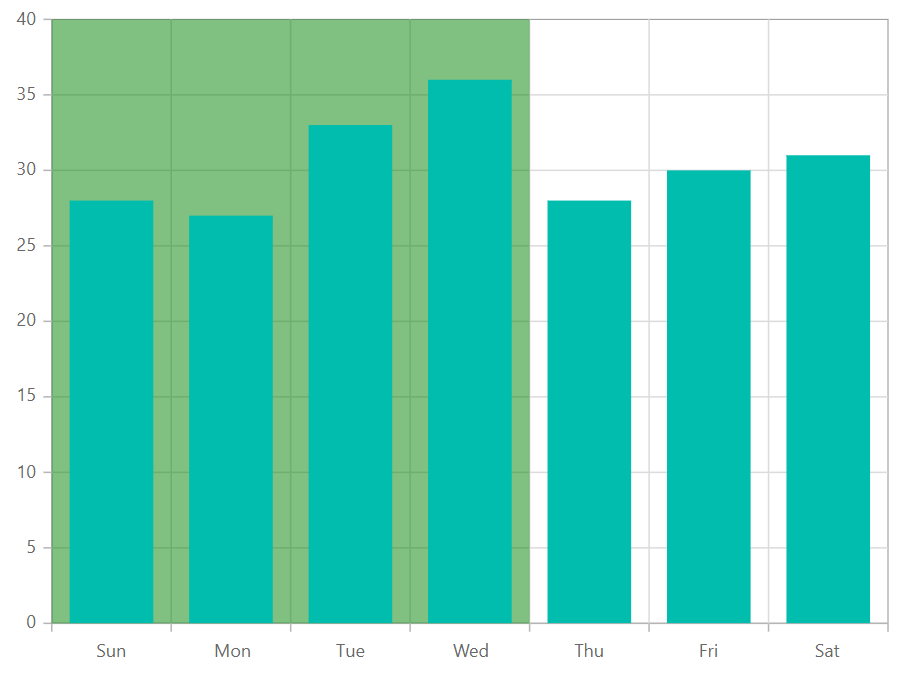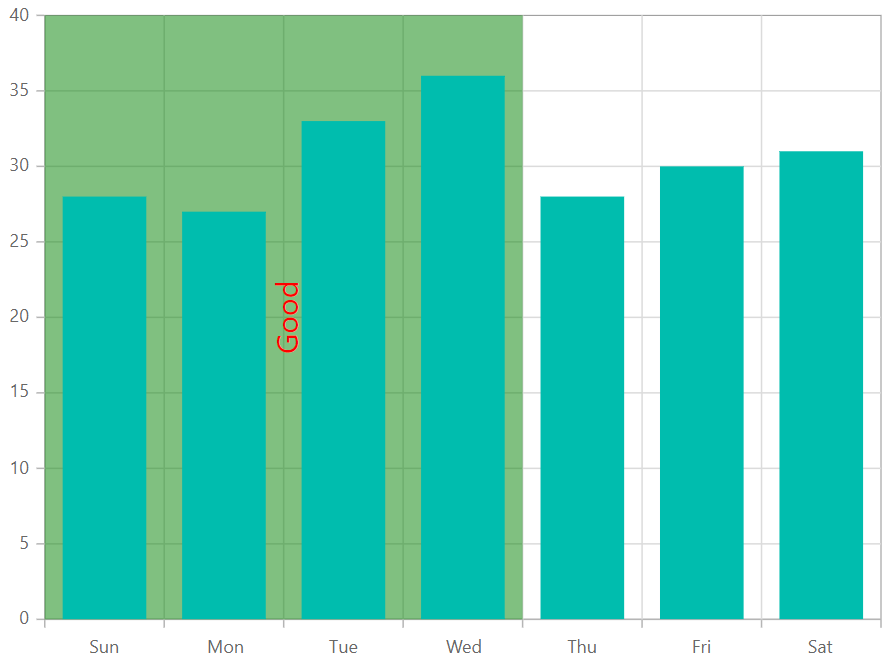# Stripline in Blazor Charts Component

10 Aug 20236 minutes to read

## Horizontal striplines

By adding the ChartStripline on the vertical axis, one can create a horizontal stripline. Striplines are drawn in the provided start-to-end range, and an axis can have multiple striplines.

``````@using Syncfusion.Blazor.Charts

<SfChart>
<ChartPrimaryXAxis ValueType="Syncfusion.Blazor.Charts.ValueType.Category"/>

<ChartPrimaryYAxis>
<ChartStriplines>
<ChartStripline Start="20" End="25" Color="red"/>
<ChartStripline Start="32" End="35" Color="blue"/>
</ChartStriplines>
</ChartPrimaryYAxis>

<ChartSeriesCollection>
<ChartSeries Type="ChartSeriesType.Column" DataSource="@WeatherReports" XName="X" YName="Y">
</ChartSeries>
</ChartSeriesCollection>

</SfChart>

@code{
public class ChartData
{
public string X { get; set; }
public double Y { get; set; }
}

public List<ChartData> WeatherReports = new List<ChartData>
{
new ChartData { X = "Sun", Y = 28 },
new ChartData { X = "Mon", Y = 27 },
new ChartData { X = "Tue", Y = 33 },
new ChartData { X = "Wed", Y = 36 },
new ChartData { X = "Thu", Y = 28 },
new ChartData { X = "Fri", Y = 30 },
new ChartData { X = "Sat", Y = 31 }
};
}``````## Vertical striplines

By adding the ChartStripline on the horizontal axis, one can create a vertical stripline. Striplines are drawn in the provided start-to-end range, and an axis can have multiple striplines.

``````@using Syncfusion.Blazor.Charts

<SfChart>
<ChartPrimaryXAxis ValueType="Syncfusion.Blazor.Charts.ValueType.Category">
<ChartStriplines>
<ChartStripline Start="2" End="3" Color="#EEFFCC" />
<ChartStripline Start="4" End="5" Color="pink" />
</ChartStriplines>
</ChartPrimaryXAxis>

<ChartSeriesCollection>
<ChartSeries Type="ChartSeriesType.Column" DataSource="@WeatherReports" XName="X" YName="Y">
</ChartSeries>
</ChartSeriesCollection>
</SfChart>

@code{
public class ChartData
{
public string X { get; set; }
public double Y { get; set; }
}

public List<ChartData> WeatherReports = new List<ChartData>
{
new ChartData { X = "Sun", Y = 28 },
new ChartData { X = "Mon", Y = 27 },
new ChartData { X = "Tue", Y = 33 },
new ChartData { X = "Wed", Y = 36 },
new ChartData { X = "Thu", Y = 28 },
new ChartData { X = "Fri", Y = 30 },
new ChartData { X = "Sat", Y = 31 }
};
}``````## Striplines customization

The Start property in a stripline can be used to customize the starting value in that stripline. The End property customizes the end value in the same way. Both Size and Border properties can be used to customize the stripline’s size and border. The ZIndex property can be used to alter the order of the stripline, determining whether it should be drawn behind or over the series elements.

``````@using Syncfusion.Blazor.Charts

<SfChart>
<ChartPrimaryXAxis ValueType="Syncfusion.Blazor.Charts.ValueType.Category">
<ChartStriplines>
<ChartStripline StartFromAxis="true" Size="4" ZIndex="ZIndex.Behind" Opacity="0.5" Color="green"/>
</ChartStriplines>
</ChartPrimaryXAxis>

<ChartSeriesCollection>
<ChartSeries Type="ChartSeriesType.Column" DataSource="@WeatherReports" XName="X" YName="Y">
</ChartSeries>
</ChartSeriesCollection>
</SfChart>

@code{

public class ChartData
{
public string X { get; set; }
public double Y { get; set; }
}

public List<ChartData> WeatherReports = new List<ChartData>
{
new ChartData { X = "Sun", Y = 28 },
new ChartData { X = "Mon", Y = 27 },
new ChartData { X = "Tue", Y = 33 },
new ChartData { X = "Wed", Y = 36 },
new ChartData { X = "Thu", Y = 28 },
new ChartData { X = "Fri", Y = 30 },
new ChartData { X = "Sat", Y = 31 }
};
}``````## Text customization

TextStyle and Rotation properties can be used to customize and rotate the text presented in a stripline. The HorizontalAlignment and VerticalAlignment properties can be used to customize the horizontal and vertical alignment of the stripline text.

``````@using Syncfusion.Blazor.Charts

<SfChart>
<ChartPrimaryXAxis ValueType="Syncfusion.Blazor.Charts.ValueType.Category">
<ChartStriplines>
<ChartStripline StartFromAxis="true" Size="4" ZIndex="ZIndex.Behind" Opacity="0.5" Color="green" Text="Good"
HorizontalAlignment="Anchor.Middle" VerticalAlignment="Anchor.Middle">
<ChartStriplineTextStyle Size="20px" Color="red"/>
</ChartStripline>
</ChartStriplines>
</ChartPrimaryXAxis>

<ChartSeriesCollection>
<ChartSeries Type="ChartSeriesType.Column" DataSource="@WeatherReports" XName="X" YName="Y">
</ChartSeries>
</ChartSeriesCollection>
</SfChart>

@code{

public class ChartData
{
public string X { get; set; }
public double Y { get; set; }
}

public List<ChartData> WeatherReports = new List<ChartData>
{
new ChartData { X = "Sun", Y = 28 },
new ChartData { X = "Mon", Y = 27 },
new ChartData { X = "Tue", Y = 33 },
new ChartData { X = "Wed", Y = 36 },
new ChartData { X = "Thu", Y = 28 },
new ChartData { X = "Fri", Y = 30 },
new ChartData { X = "Sat", Y = 31 }
};
}``````NOTE

Refer to our Blazor Charts feature tour page for its groundbreaking feature representations and also explore our Blazor Chart Example to know various chart types and how to represent time-dependent data, showing trends at equal intervals.